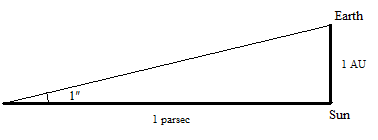# Thread: AU's, parsecs, and light years

1. I am sure I could easily look up a conversion calculator on the net and find this answer easy, but I'd like to work my way through this problem.

Ch 1, Problem 57
An astronomical unit (AU) is equal to the average distance from Earth to the Sun, about 92.9 × 106 mi. A parsec (pc) is the distance at which a length of 1 AU would subtend an angle of exactly 1 second of arc (Figure 1-9). A light-year (ly) is the distance that light, traveling through a vacuum with a speed of 186000 mi/s, would cover in 1.0 year. Express the EarthSun distance in (a) parsecs and (b) light-years.My work:
I see from the diagram, that the substended section of AU forms the base of an isosceles triangle. We can, of course, divide our isosceles triangle in half to give us an easy to work with right triangle with a base of (1/2)AU, and an apex angle (incorrect term?) of (1/2) second, or (1/7200) degree. To find pc, I reasoned that:However, since we want to find out how many AU's there are in a pc, we can divide out the AU and get:The answer I get is a nice neat looking:However this does not seem to be the correct answer.

As for the light years, I seem to have gotten the correct answer by converting the speed of light in a second to the speed of light in a year, then dividing AU by this number to get 1.58270073*10^(-5) light years.2.

3. Are you sure youve drawn the diagram correctly? I thought it should bewhich should give..5 * cosecant((1/7200) degrees) = 206 264.806

and

1 Parsec = 206 264.806 Astronomical Units

So I think you just made a calculation error. You used radians instead of degrees didn't you.5. That was it exactly. I'll have to be more careful with radians/degrees.

Yes, the answer I get is 206264.8062

And of course the problem asks for the Earth-Sun distance in parsecs, so the inverse of 206264.8062 gives the correct answer of 0.000004848136811 parsecs.Bookmarks
 Posting Permissions
 You may not post new threads You may not post replies You may not post attachments You may not edit your posts   BB code is On Smilies are On [IMG] code is On [VIDEO] code is On HTML code is Off Trackbacks are Off Pingbacks are Off Refbacks are On Terms of Use Agreement